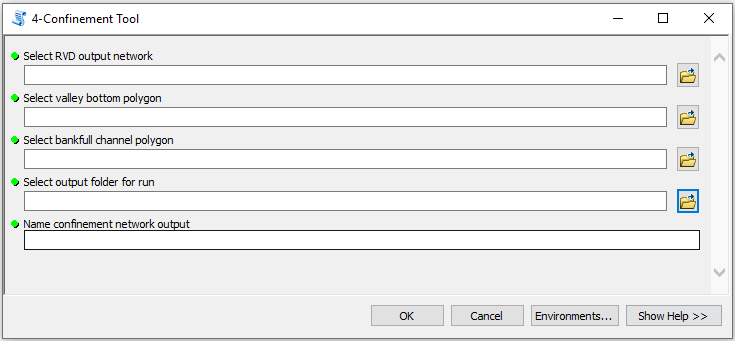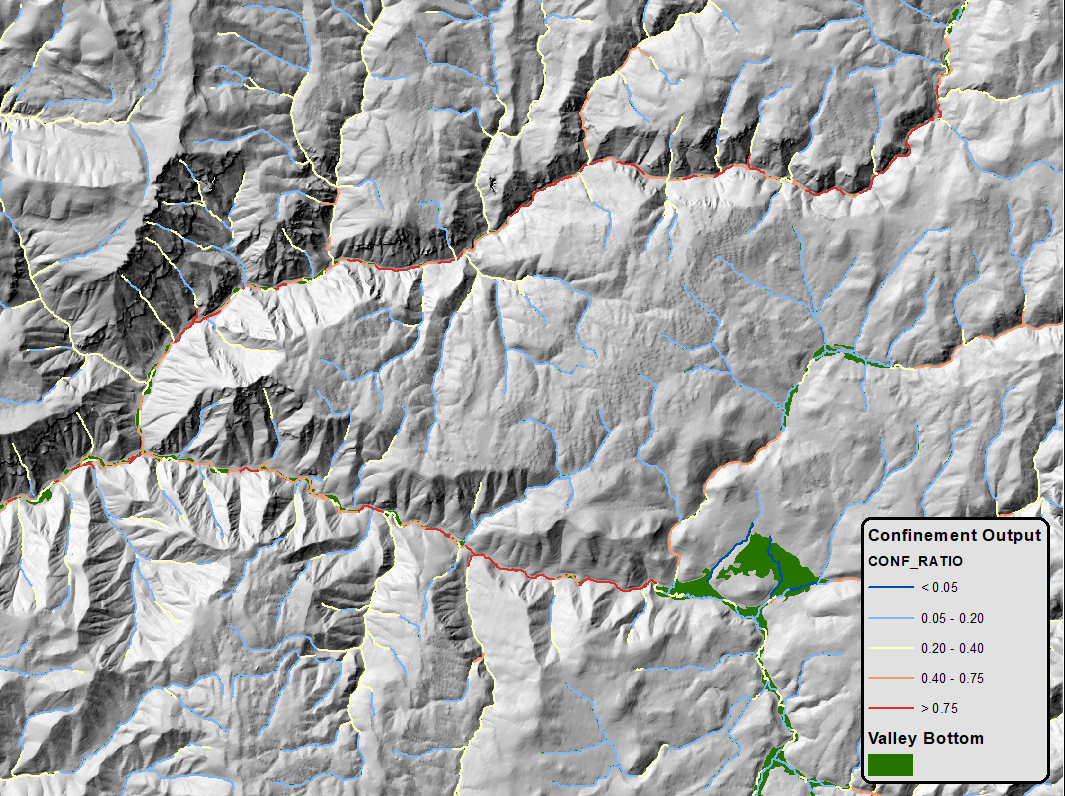# Step 5 - Confinement Tool

The Confinement Tool calculates an index of confinement by dividing the bankfull channel width by the valley bottom width at each reach. The output from this tool is needed to run the Riparian Condition Assessment Tool

## Parameters• Select RVD network: Select output shapefile from the RVD tool.
• Select valley bottom polygon: Select input valley bottom polygon.
• Select bankfull channel polygon: Select polygon output from the Bankfull Channel tool.
• Select output folder for run: Output folder for this run of RCAT, where intermediates and outputs will be saved, in format “Outputs/Output_01”.
• Name confinement network output: Name for output network including confinement fields.

NOTE: The confinement tool relies heavily on accurate bankfull channel and valley bottom inputs. These inputs should be cross-verified using aerial imagery/basemaps or field knowledge before running this tool.

## Outputs

### Attribute Fields

• `BFC_Area`: Area of the bankfull channel polygon corresponding to the given stream segment.
• `VAL_Area`: Area of the valley bottom polygon corresponding to the given stream segment.
• `BFC_Width`: Averge width of the bankfull channel polygon corresponding to the given stream segment, calculated as `BFC_Area` / `Rch_Len`.
• `VAL_Width`: Average width of the valley bottom polygon corresponding to the given stream segment, calculated as `VAL_Area` / `VAL_Width`.
• `Rch_Len`: Reach length, calculated as the lenght of the given stream segment.
• `CONF_RATIO`: Confinement index, calculated as `BFC_Width` / `VAL_Width`. Higher values indicate more confined areas where the bankfull channel width is close to the valley bottom width, while lower values indicate less confined areas where the bankfull channel is narrower than the valley bottom.

### Confinement Network

Output stream network including the above attribute fields, symbolized by the confinement index `CONF_RATIO`:## Confinement Tool Workflow

1. Thiessen polygons are created based on segment midpoints.
2. Valley bottom polygons and bankfull channel polygons are created by clipping the thiessen polygons to the valley bottom or bankfull channel.
3. The area of each valley bottom polygon `VAL_Area` is calculated and then joined to the corresponding stream segment.
4. The area of each bankfull channel polygon `BFC_Area` is calculated and then joined to the corresponding stream segment.
5. The length of each segment `Rch_Len` in the stream network is calculated.
6. The average width of the bankfull channel polygon `BFC_Width` corresponding to each stream segment is calculated by dividing the polygon area `BFC_Area` by the segment length `Rch_Len`. This value essentially is the width of the bankfull channel polygon converted to a rectangle of equal area.
7. The average width of the valley bottom polygon `VAL_Width` corresponding to each stream segment is calculated by dividing the polygon area `VAL_Area` by the segment length `Rch_Len`. This value essentially is the width of the valley bottom polygon converted to a rectangle of equal area.
8. The confinement index `CONFIN_RATIO` is calculated by dividing the bankfull channel width `BFC_Width` by the valley bottom width `VAL_Width`.